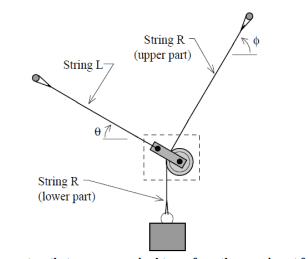# Finding the force on a cord from a pulley

• Johno123
In summary, the student is trying to find the 'String R (lower part)' force. They are asking for help with this question, and have tried to do some calculations themselves. It is difficult to tell where they are having trouble, so they ask for help. If it is not homework, the student would like to see a free body diagram of the pulley.f

#### Johno123

(Mentor note: moved from General Eng hence not homework template)This is the given scenario. I'm trying to find the 'String R (lower part)' force. I know every other variable (the angles, string L and string R and the Rectangle is a mass m). Any help would be appreciated!

#### Attachments

Last edited by a moderator:
Is that a mass m hanging from String R?

•CWatters and Johno123
Is that a mass m hanging from String R?

Yes sorry I forgot to put that in! Just fixed it.

Yes sorry I forgot to put that in! Just fixed it.
Hi again.
If this is homework, we have a section called Homework Help, to which it can be moved.
Is it homework?

It has an outline to follow, which gives you a much better responses from people who will guide you to a solution.

Hi again.
If this is homework, we have a section called Homework Help, to which it can be moved.
Is it homework?

It has an outline to follow, which gives you a much better responses from people who will guide you to a solution.

No its not homework. I'm doing some study ahead for my course next year and I wanted some help with this question.

No its not homework. I'm doing some study ahead for my course next year and I wanted some help with this question.
So what have you tried so far?
It is difficult to tell where you are having trouble without showing some of your calculations and equations.

I'm trying to find the 'String R (lower part)' force.
That's confusing to me.
It looks like a trivial question because string R's lower segment just holds up mass M.
"force on a cord from a pulley"

If nothing is in motion that seems straightforward to find.

Have you drawn a free body diagram of that pulley showing the forces on it? Since nothing is moving you can make believe everything is glued together.

Is that a mass m hanging from String R?
That was probably a hint rather than a question.

•scottdave
It like be interesting to see the whole problem statement.

Do you know how the tension in string R in the upper part compares with the lower part? If you are studying ahead, this is an important part of your learning.

•jim hardy